• Submissions# On Soliton Solutions of the Drinfeld-Sokolov- Wilson System by He’s Variational Principle

Mohammed K Elboree*

Department of Mathematics, Egypt

*Corresponding author:Mohammed K Elboree, Department of Mathematics, Egypt

Submission: November 29, 2018; Published: April 4, 2019

DOI: 10.31031/EME.2019.02.000543ISSN 2640-9690
Volume2 Issue4

#### Abstract

The aim of this paper is to obtain the traveling wave solutions for the Drinfeld-Sokolov-Wilson system by He’s semi-inverse variational principle which includes the solitary and periodic wave solutions by a suitable choice for the parameters. We analysis these solutions physically by some gores to complement this study. Finally, this method can be used successfully for solving integrable and nonintegrable equations.

Keywords: He’s semi-inverse variational principle; Traveling wave solutions; Dronfield-Sokolov-Wilson system; Soliton solutions; Periodic wave solutions

#### Introduction

Nonlinear partial differential equations (NLPDEs) is used to describe many important phenomena in mathematical physics, mechanics, chemistry, biology, etc. Such as Cortège de Vries equation, Burgers equation, Schrodinger equation, Bossiness equation and so on. So, the discovery of the exact solutions of nonlinear partial differential equations is of the most important priorities. Many effective methods are used to construct traveling wave solutions of NLPDEs, among of these methods, Adomian decomposition method , the homotropy perturbation method , the variational iteration method [3, 4], the He’s variational approach , the extended homoclinic test approach [6, 7], homogeneous balance method [8-11], Jacobi elliptic function method [12-15], Baclund transformation [16, 17], G0=G expansion method  . He’s semi-inverse variational principle is used to obtain the traveling wave solutions for Dronfield-Sokolov-Wilson system which include the solitary and periodic wave solutions by a suitable choice for the parameters.

It is important to point out that a new constrained variational principle for heat conduction is obtained recently via the semi-inverse method combined with separation of variables, which is an exactly the same with He-Lee’s variational principle a short remark on the history of the semi-inverse method for establishment of a generalized variational principle is given in . In soliton theory, we aim to search for the solitary wave solutions for NLPDEs using various methods . In particular, we used in this paper the variational principle, which enables us to and the Lagrangian for the Drinfeld-Sokolov-Wilson system, which related to the conservation laws which plays an important role in solution process [23,24] and provides physical insight into the nature of the solution of this problem as shown by gures. Also, this method helps in establishing connections between the physics and mathematics and much more active than the Noether’s theorem [25, 26].

The key idea in this paper is to use the He’s semi-inverse variational principle to and out several exact solutions for Drinfeld-Sokolov-Wilson system. Conclusions is given in the last of this paper.

#### Methodology

Suppose we are given a nonlinear partial differential equations (NLPDEs) in the following form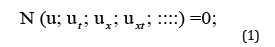where x and t are the independent variables.

This method can be summarizing as follows

A. By using the wave solutionswe can transform Eq. (1)

into an ordinary differential equation (ODE)

N(U; cU ; U ; cU ; :::) = 0

B. Integrating Eq. (2) and setting the integration constants equal to zero for simplicity.

C. Construct the Lagrangian in the following form according to the He’s semi-inverse method,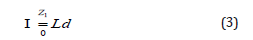where L is a Lagrangian for the Eq. (2)

D. By a Ritz method , one can obtain a different forms of solitary wave solutions, such as

U( )= Asech (B); U( ) = Acsch (B); U( ) = A tanh (B); ss

and so on. In this paper, we concentrate to obtain a solitary wave solution in the form

U( )= Asech (B) (4)

where A and B are constants to be determined.

Substituting Eq. (4) into Eq. (3) and making I stationary with respect to A and B results in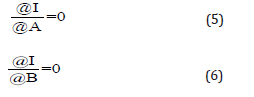We can obtain A and B by solving Eqs. (5) and (6). Therefore, we can construct the solitary wave solution for Eq. (4)

#### Soliton solutions to Drinfeld-Sokolov-Wilson system

We aim in this section to obtain the soliton solutions for the Drinfeld-Sokolov-Wilson system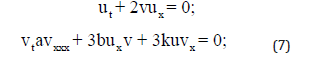where a; b and k are constants .

By using the transformation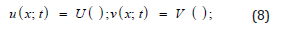where = x ct + 0, c; 0 are constants.

Substituting Eq. (8) into Eq. (7), we and thatwhere the prime denotes to the derivative with respect to the variable a, b, c and k are constants. Integrating Eqs. (9), we obtain the relation between the variables V (), U () as followsSubstituting V () in Eqs. (9), we obtain after integration and setting the constant of integration zero for simplicityAccording to , by He’s semi-inverse variational principle , we can obtain the following variational formulationAccording to the Ritz-like method, we search a solitary wave solution in the form

V ( ) = Asech (B ) (13)

Substituting Eq. (13) into Eq. (12), we have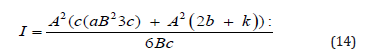To and the constants A and B, we solve the following equationsFrom Eqn. (15), we getTherefore, the solitary wave solutions for Drinfeld-Sokolov- Wilson system constructed as follows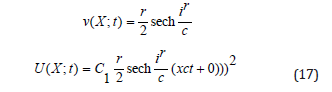For a suitable choice for a parameter in Eq. (17), Figure 1 & 2 describes the shape of solitons for waves u (x; t) and v (x; t) respectively (Figure 1)

Figure 1:The soliton solution of Eq. (17).Figure 2:The soliton solution of Eq. (17).For another constant A and B in Eq. (16) (Figure 2)

We search another soliton solution in the form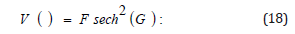where F and G are constants to be determined. Substituting Eq. (18) into Eq. (12), we obtainTo and the constants F and G, we solve the following equations (19)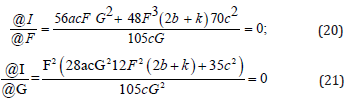From Eqn. (20), we getFor a suitable choice for a parameter in Eq. (22), Figure 3 & 4 describes the shape of solitons for waves u (x; t), and v (x; t) respectively for another constant F and G in Eq. (21)

Figure 3:The soliton solution of Eq. (22).Figure 4:The soliton solution of Eq. (22).#### Physical Discussions

In this section, we discusses the physical explanation of the solutions obtained for the Drinfeld-Sokolov-Wilson system (7), namely (17), (22) which are soliton pattern solutions caused by the delicate balance between nonlinearity effect V3 and dispersion effect V1000 which have an infinite tails and keep their form and velocity after an entire interaction with others solitons. We display the time evolution of these solutions by some gores to describe the dynamical properties in 3D graphs in Figure 1-4 are the forth solutions (22).

We note the wave speed c acts a critical role in a physical structure of the solutions for Drinfeld-Sokolov-Wilson system. The 3D graphs in Figure 1-4 show the shape of propagation of soliton solutions (17) and (22) for the Drinfeld-Sokolov-Wilson system (7).

#### Result and Discussion

He’s semi-inverse variational principle was used to obtain the traveling wave solutions for Drinfeld-Sokolov-Wilson system including new type of solitary wave solutions. He’s variational principle is a very dominant instrument to and the soliton solutions for various nonlinear equations in mathematical physics and may be important for the explanation of some practical physical problems. This method is a powerful mathematical tool for solving other nonlinear evolution equations arising in mathematical physics. This method makes the underlying idea clear and not darkened by the unnecessarily by complicated form of mathematical expression. Also, we can combine the well-known method such as Jacobi elliptic function method and exp-function method with He’s semi-inverse variational method, but they need a mathematical software, and this method is extreme simplicity and concise results for a wide range of NLPDEs compared with the Noether’s theorem.

© 2019 Mohammed K Elboree. This is an open access article distributed under the terms of the Creative Commons Attribution License , which permits unrestricted use, distribution, and build upon your work non-commercially.

We at Crimson Publishing are a group of people with a combined passion for science and research, who wants to bring to the world a unified platform where all scientific know-how is available read more...